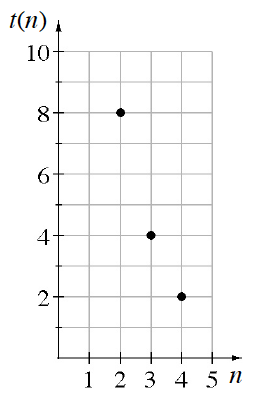### Home > INT1 > Chapter 6 > Lesson 6.2.3 > Problem6-77

6-77.

Write an explicit equation for the sequence graphed at right.

The multiplier for this graph is $\frac{1}{2}$

Work backward to find term $0$, or the starting value.

Use the starting value and the multiplier to write the equation.

$t(n)=32\left( \frac{1}{2} \right)^n$